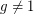Importance: High ✭✭✭
 Author(s):
 Subject: Theoretical Computer Science » Complexity
 Keywords: discrete log NP
 Posted by: cplxphil on: September 27th, 2008

Ifis prime and, we writeifsatisfies. The problem of finding such an integerfor a given(with) is the Discrete Log Problem.

Conjecture   There does not exist a polynomial time algorithm to solve the Discrete Log Problem.

The Discrete Logarithm Problem is a critical problem in number theory, and is similar in many ways to the integer factorization problem. If it were possible to compute discrete logs efficiently, it would be possible to break numerous thought-to-be unbreakable cryptographic schemes. However, although most mathematicians and computer scientists believe that the DLP is unsolvable, this conjecture is difficult to establish, because such a proof would imply that P != NP...which is the most difficult open problem in theoretical computer science.

Avi Wigderson has shown that there is no natural proof'' (in the sense of [RR]) that the DLP requires circuits of greater than half-exponential size. The key idea is that a natural proof that the DLP is hard would yield a method for breaking discrete-log-based cryptosystems, and this is a contradiction. Of course, it could still be that DLP is provably hard, but by a proof that is not natural.''

## Bibliography

[RR] Alexander A. Razborov and Steven Rudich, Natural proofs, Journal of Computer and System Sciences 55 (1997), 24–35.

* indicates original appearance(s) of problem.